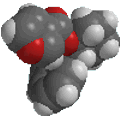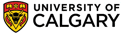﻿R/S nomenclatureBasic IUPAC Organic Nomenclature

R- and S- nomenclature of chirality centers

The Cahn-Ingold-Prelog priority rules are used for naming chirality centers and geometric isomers (e.g. E- or Z-alkenes)

These rules are used to establish the priority of the groups attached to the chirality center and are based on atomic number, and the first point of difference.

In the simplest and most common case, a chirality center is characterised by an atom that has four different groups bonded to it in such a manner (e.g. tetrahedral) that it has a non-superimposable mirror image.  Terms such as an asymmetric, stereogenic or chiral center have been used in the past.

 The most common chirality center in organic chemistry is a carbon atom with four different groups attached

In order to assign the configuration as R or S:
• Identify each of the chirality centers (most commonly an sp3 C with 4 different groups attached)
Then at each chirality center....
• Assign the priority (high = 1 to low = 4) to each group attached to the chirality center based on atomic number.
• Reposition the molecule so that the lowest priority group is away from you as if you were looking along the C-(4) σ bond. If you are using a model, grasp the lowest priority group in your fist.
• Determine the relative direction of the priority order of the three higher priority groups (1 to 2 to 3)
• If this is clockwise then it is the R-stereoisomer (Latin; rectus = right handed)
• If this is counter-clockwise then it is the S-stereoisomer (Latin; sinister = left handed)
• If there is more than one stereocenter, then the location needs to be included with the locant, e.g. (2R)-
Subrules:
• Isotopes: H vs D ? Since isotopes have identical atomic numbers, the mass number is used to discriminate them so D > H
• If the same atom is attached, then look for the first point of difference by moving out one atom at a time, locate the first point of difference and apply rules there.
• If a multiple bond is encountered, treat it as if the atoms are attached by the same number of single bonds e.g. C=C is treated a 2 C-C and C=O is 2 C-O

HINT:

• at each center, list out the 3 new atoms attached as you move away from the chirality center (see the 2nd example shown below)
• list these groups in their priority order (i.e. high to low atomic number)
• use this "list" to locate the first point of difference

Example:  chlorofluoroiodomethane

 The chirality center should be easy to spot, and the four attached groups are in priority order, highest to to lowest: I (purple), Cl (green), F(brown) and H (white) Rotate the image on the left so the you are looking along the C-H bond and the H is away from you, then determine the direction of high to low priority.  Since it decreases clockwise, this is the R configuration.starting point identify the chirality center(s assign the relative priorities then rotate the low priority group away (to the back) determine the sense of groups 1 - 3 clockwise = R

Example: cyclohex-2-enolstarting point identify the chirality center(s) and show the implied H atom assign the relative priorities.... O > C > C > H Because we have two C groups we need to list the groups the C are attached to in atomic number order (note how the multiple bond is handled) the first point of difference is the C > H so the C group on the left is the higher priority with the low priority group already at the back, determine the sense of groups 1 - 3 counterclockwise = S©Dr. Ian Hunt, Department of Chemistry